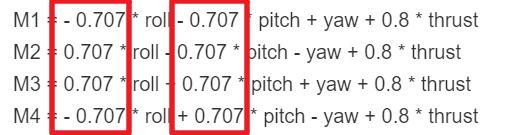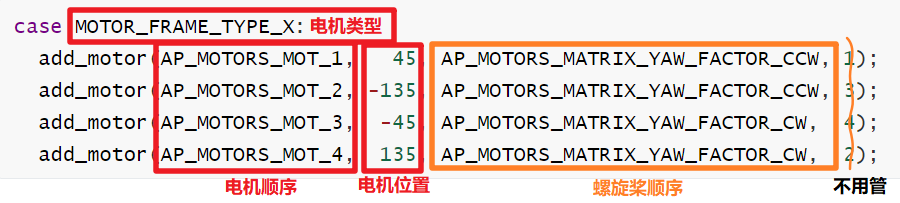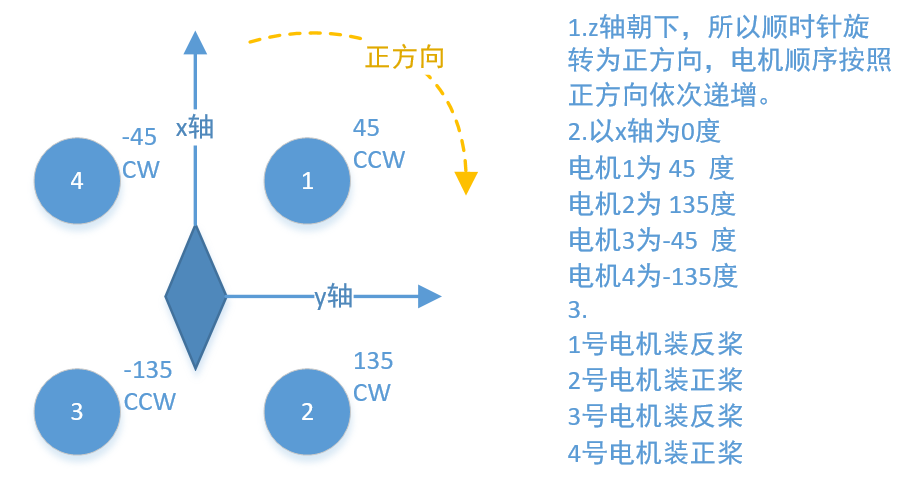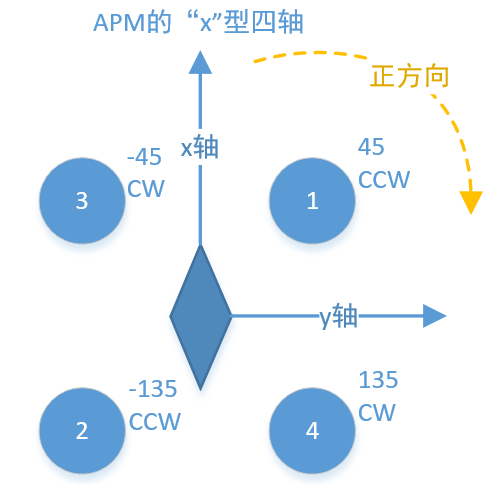[飞控] 手把手教你修改控制分配矩阵libraries\AP_Motors\AP_MotorsMatrix.cpp 405

case MOTOR_FRAME_TYPE_X:
add_motor(AP_MOTORS_MOT_1,   45, AP_MOTORS_MATRIX_YAW_FACTOR_CCW, 1);
add_motor(AP_MOTORS_MOT_2, -135, AP_MOTORS_MATRIX_YAW_FACTOR_CCW, 3);
add_motor(AP_MOTORS_MOT_3,  -45, AP_MOTORS_MATRIX_YAW_FACTOR_CW,  4);
add_motor(AP_MOTORS_MOT_4,  135, AP_MOTORS_MATRIX_YAW_FACTOR_CW,  2);

libraries\AP_Motors\AP_MotorsMatrix.cpp

void AP_MotorsMatrix::add_motor(int8_t motor_num, float angle_degrees, float yaw_factor, uint8_t testing_order)
{
add_motor(motor_num, angle_degrees, angle_degrees, yaw_factor, testing_order);
}

libraries\AP_Motors\AP_MotorsMatrix.cpp

void AP_MotorsMatrix::add_motor(int8_t motor_num, float roll_factor_in_degrees, float pitch_factor_in_degrees, float yaw_factor, uint8_t testing_order)
{
motor_num,                                  //电机序号
cosf(radians(roll_factor_in_degrees + 90)), //电机在roll方向的系数
yaw_factor,                                 //电机在yaw方向的系数
testing_order);                             //测试序号 不用管
}

roll_factor，pitch_factor，yaw_factor。0.飞机的坐标系（隐藏条件）

1.飞机类型

2.螺旋桨的旋转方向

ok，让我们手把手开始吧。

1.定义机体坐标系

2.定义飞机的机型

3.定义螺旋桨的旋转方向

ok我们的飞机就设计完了，长这样。ok，让我们把这些关键系数填进函数

case 我的飞机:
add_motor(1号电机,   45, 反桨CCW, 没什么用的序号1);
add_motor(2号电机,  135, 正桨CW,  没什么用的序号2);
add_motor(3号电机, -135, 反桨CCW, 没什么用的序号3);
add_motor(4号电机,  -45, 正桨CW,  没什么用的序号4);
//add_motor(AP_MOTORS_MOT_1,   45, AP_MOTORS_MATRIX_YAW_FACTOR_CCW, 1);
//add_motor(AP_MOTORS_MOT_2,  135, AP_MOTORS_MATRIX_YAW_FACTOR_CCW, 2);
//add_motor(AP_MOTORS_MOT_3, -135, AP_MOTORS_MATRIX_YAW_FACTOR_CW,  3);
//add_motor(AP_MOTORS_MOT_4,  -45, AP_MOTORS_MATRIX_YAW_FACTOR_CW,  4);

APM的“x”型四轴:

case MOTOR_FRAME_TYPE_X:
add_motor(AP_MOTORS_MOT_1,   45, AP_MOTORS_MATRIX_YAW_FACTOR_CCW, 1);
add_motor(AP_MOTORS_MOT_2, -135, AP_MOTORS_MATRIX_YAW_FACTOR_CCW, 3);
add_motor(AP_MOTORS_MOT_3,  -45, AP_MOTORS_MATRIX_YAW_FACTOR_CW,  4);
add_motor(AP_MOTORS_MOT_4,  135, AP_MOTORS_MATRIX_YAW_FACTOR_CW,  2);